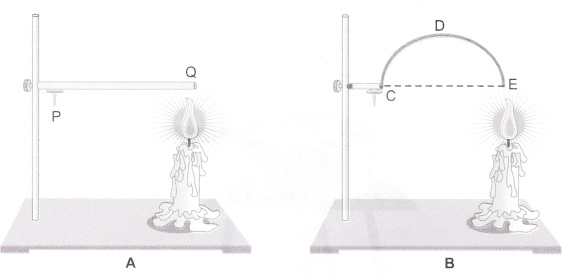# Look at figures given below. The length of wire PQ in case of A is equal to the diameter of the semicircle formed by the wire CDE, in case B

Look at figures given below.The length of wire PQ in case of A is equal to the diameter of the semicircle formed by the wire CDE, in case B. One pin is attached to each wire with the help of wax as shown in figure above. Which pin will fall first? Explain.